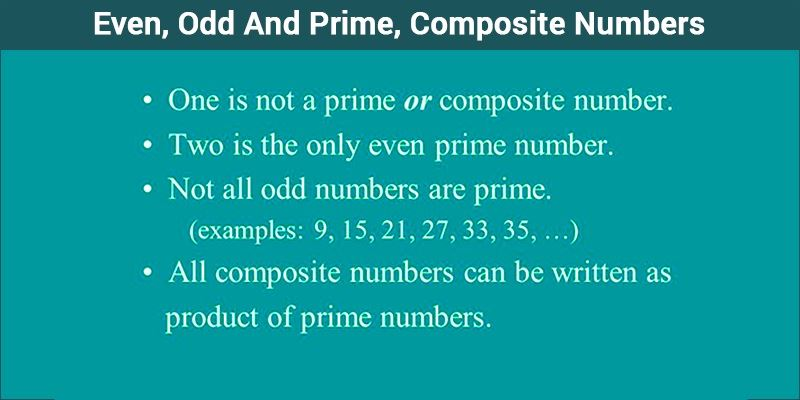# Prime, Composite And Even, Odd Numbers

A life without numbers is difficult. Numbers influence our daily routine in such a way that almost everything we handle depends on numbers. The mobile numbers, time, money, dates, years and so on. Numbers can be of different types, such as integers, natural numbers, whole numbers, odd or even numbers, prime numbers, composite numbers, rational numbers etc. Here, two different classes of numbers are explained. They are odd or even numbers and another class is prime or composite numbers.## Even and Odd Numbers in Maths

Integers are a set of numbers which do not have a fractional part, they can be positive, negative or zero.

Ex: …, -3, -2, -1, 0, 1, 2, 3,….

Definition of Even Numbers:

Integers which are divisible by 2 are known as even numbers. In other words, numbers whose unit place is 0, 2, 4, 6, 8 are divisible by 2 and such numbers are even numbers. Even numbers leave no remainder (i.e. remainder is 0) when divided by 2.

Ex: 8, 14, 16, 50, 62,…

Definition of Odd Numbers:

In contrast, to even numbers, integers which are not divisible by 2 are known as odd numbers. They have 1, 3, 5, 7, 9 at their unit place. Odd numbers leave remainder 1 when divided by 2.

Ex: 13, 21, 65, 77, 49,…

Also, check: Odd numbers

Even numbers can be positive or negative. In case of negative, we may call them as negative even integers, whereas positive numbers as positive even integers. Same concepts is applicable for odd numbers also.

### General Representation of Odd and Even numbers

In general format, every Even number can be written in the form of (2n), whereas, every odd number can be written in the form of (2n-1) or (2n + 1), where n is a natural number. In case of integers, these can be represented as 2k and 2k + 1 or 2k – 1, where k is any integer.

#### Example of even integers:

-16, -8, 2, 56, 200

Here,

-16 = 2 × (-8)

-8 = 2 × (-4)

2 = 2 × 1

56 = 2 × 28

200 = 2 × 100

#### Example of odd integers:

-59, -17, -5, 3, 53, 1, 555,-601

Here,

-59 = 2 × (-30) + 1 = 2 × (-29) – 1

-17 = 2 × (-9) + 1 = 2 × (-8) – 1

-5 = 2 × (-3) + 1 = 2 × (-2) – 1

3 = 2 × (1) + 1 = 2 × (2) – 1

53 = 2 × (26) + 1 = 2 × (27) – 1

1 = 2 × (0) + 1 = 2 × (1) – 1

555 = 2 × (277) + 1 = 2 × (278) – 1

-601 = 2 × (-301) + 1 = 2 × (-300) – 1

## Prime and Composite Numbers in Maths

Definition of Prime Numbers:

A natural number which has exactly two factors, i.e.  1 and the number itself, is a prime number. In simple words, if a number is only divisible by 1 and itself, then it is a prime number.  Every prime number is an odd number except number 2.

#### For example:

2, 3, 5, 7, 11, 19, 37, 41, 313, 241 etc.

2 = 1 × 2

3 = 1 × 3

5 = 1 × 5 and so on.

Thus, all the above mentioned numbers have exactly two factors, i.e. 1 and the number itself. Hence, they are prime numbers.

Definition of Composite Numbers:

Every non-prime number is a composite number. Composite numbers are those natural numbers which have more than two factors. Such numbers are divisible by other numbers as well.

#### For example:

4, 6, 8, 10, 12, 14, 500, 6000  etc.

These numbers can be written as:

4 = 1 × 4, 4 = 2 × 2

6 = 1 × 6, 6 = 2 × 3

8 = 1 × 8, 8 = 2 × 4

10 = 1 × 10, 10 = 2 × 5

12 = 1 × 12, 12 = 2 × 6, 12 = 3 × 4 and so on.

Thus, all the above mentioned numbers have more than two factors, i.e. other than 1 and the number itself. Hence, they are composite numbers.

Some facts about prime numbers and composite numbers are:

• 1 is neither a prime nor a composite number.
• The only even number which is a prime is 2. It is the smallest prime number.
• Except 2, all other prime numbers are odd numbers.

## Video Lesson on Prime NumbersStay tuned with BYJU’S to learn more about prime numbers, even & odd number and many more.

Quiz on Prime Composite and Even Odd

1. I love the information😍

2. It’s very useful for us.

3. Hi I like the byjus

4. thank you so much Byjus helped a lot for BNAT

5. Thank you very much for the lesson

6. thank you..its useful..

7. Which number is prime and also even

• The only even number which is a prime is 2. It is the smallest prime number. Except 2, all other prime numbers are odd numbers.